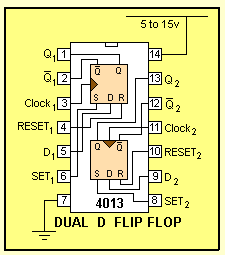CD 4013The CD 4013 is a DUAL FLIP FLOP. The CD 4013 is a  CMOS chip.  Minimum supply voltage 6v Maximum supply voltage 15v Max current per output 15mA Maximum speed of operation 5MHz

Circuits built around a CD 4013:
The CD 4013 can be used as a frequency divider in which the output is half the input frequency.
It can also be used in TOGGLE MODE (this is exactly the same as a frequency divider) where the output toggles ON then OFF after each clock (input pulse).
This is exactly the same as a FLIP FLOP or MEMORY CELL.
The following circuit shows two flip flops creating a "divide-by-four."
The output Q is taken to Data input. Note: this did not work for a Philips surface-mount CD4013BT IC. The output Q had to be delayed with a 2k2 and 1u electrolytic as shown in the second diagram.